Next: Circular Current Loop Up: Magnetostatic Fields Previous: Biot-Savart Law

# Continuous Current Distribution

Making use of the fact that the magnetic fields generated by different current loops are superposable (see Section 1.2), Equation (614) can easily be generalized to deal with the magnetic field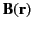generated by a continuous current distribution of current density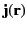. In fact,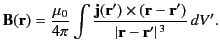(616)

For the case of a steady (i.e.,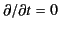) current distribution, the charge conservation law (7) yields the constraint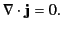(617)

Given that [see Equation (153)](618)

Equation (617) can also be written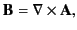(619)

where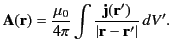(620)

Here,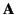is termed the vector potential. (See Section 1.3.) It immediately follows that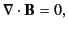(621)

which is the second Maxwell equation. (See Section 1.2.) Now,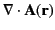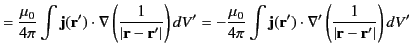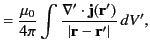(622)

where we have integrated by parts, and neglected surface terms. Thus, according to Equation (618),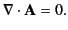(623)

In other words, the vector potential defined in Equation (621) automatically satisfies the time independent version of the Lorenz gauge condition, (13). Finally,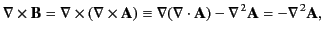(624)

where use has been made of Equations (620) and (624). It follows from Equations (621) and (25) that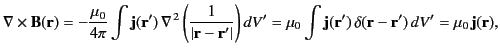(625)

or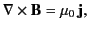(626)

which is the time independent form of the fourth Maxwell equation. (See Section 1.2.) The integral version of the previous equation, which follows from the curl theorem, is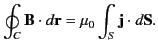(627)

This result is known as Ampère's law. Here,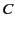is a closed curve spanned by a general surface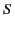.Next: Circular Current Loop Up: Magnetostatic Fields Previous: Biot-Savart Law
Richard Fitzpatrick 2014-06-27